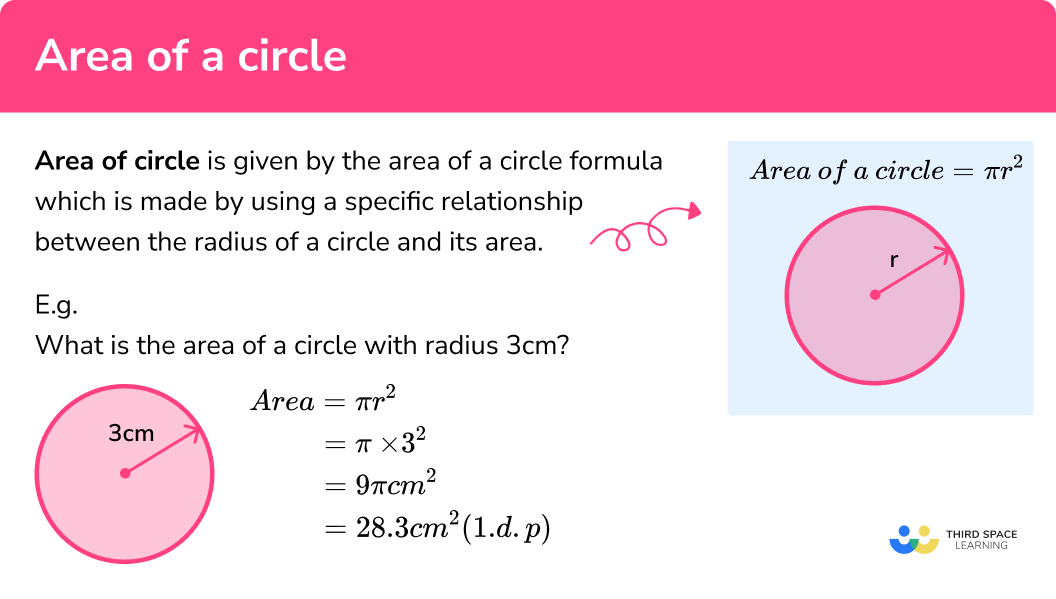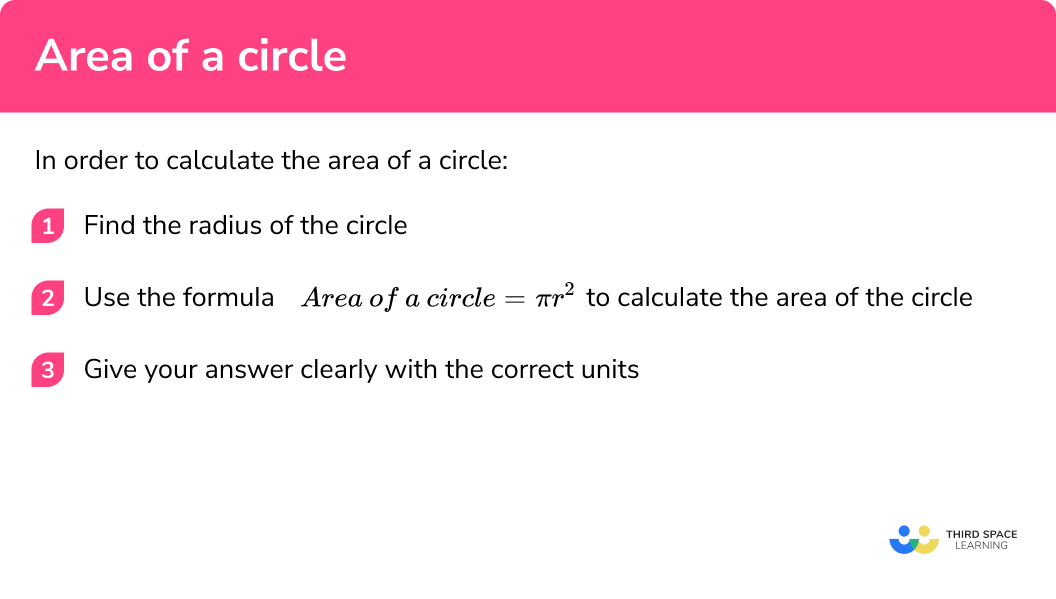# Area Of A Circle

Here we will learn about calculating the area of a circle including how to calculate the area of a circle given the radius, how to calculate the area of a circle given the diameter and how to calculate the area of a circle given the circumference.

There are also area of a circle worksheets based on Edexcel, AQA and OCR exam questions, along with further guidance on where to go next if you’re still stuck.

## What is the area of a circle?

The area of a circle is given by the area of a circle formula which is made by using a specific relationship between the radius of a circle and its area.

Area of a circle formula:

\pi \times r\times r

we usually simplify this to

E.g.
What is the area of a circle with radius 3cm ?

\begin{aligned} \text { Area } &=\pi r^{2} \\\\ &=\pi \times 3^{2} \\\\ &=9 \pi \mathrm{cm}^{2} \\\\ &=28.3 \mathrm{~cm}^{2}(1 . \mathrm{d} . \mathrm{p}) \end{aligned}

### What is the area of a circle?### What is pi?

\pi (pronounced pi) represents the ratio of the circumference of a circle to its diameter.
For all circles if you divide the length of the circumference by the length of the diameter you get the value \pi .

Note: \pi is an irrational number which means it cannot be written as a fraction. It is a non recurring decimal and has an approximate value of 3.14159…

In GCSE you should use the \pi button on your Casio calculator when working with \pi .
To get this you need to press [SHIFT][ \times 10^x ].
Alternatively you can use the value 3.142 instead.

Sometimes a question may ask you to leave an answer in terms of pi
E.g
6 \times \pi = 6\pi (this is an answer in terms of pi)

8 \times \pi = 8\pi (this is an answer in terms of pi)

10 \times \pi =  31.41... (this is an answer not in terms of pi)

## How to calculate the area of a circle

In order to calculate the area of a circle:

1. Find the radius of the circle.
2. Use the formula \text{Area of a circle} = \pi r^2 to calculate the area of the circle.

### Explain how to calculate the area of a circle## Area of a circle examples

### Example 1: calculating the area of the circle given the radius

A circle has a radius of 6cm , calculate its area.

1. Find the radius of the circle.

The radius is given in the question

2Use the formula \pi r^2 to calculate the area of the circle.

\begin{aligned} &\pi r^2\\\\ &= \pi \times r\times r\\\\ &= \pi \times6\times 6\\\\ &= 36\pi \\\\ &= 113.0973355... \end{aligned}

113.10cm^2

### Example 2: calculating the area of the circle given the diameter

A circle has a diameter of 10mm. , calculate its area.

Find the radius of the circle.

Use the formula \pi r^2 to calculate the area of the circle.

### Example 3: calculating the area of the circle, given the radius, answer in terms of 𝝅

A circle has a radius of 8m. , calculate its area.

Find the radius of the circle.

Use the formula \pi r^2 to calculate the area of the circle.

### Example 4: calculating the area of the circle given the diameter

A circle has a diameter of 420km. , calculate its area.

Find the radius of the circle.

Use the formula \pi r^2 to calculate the area of the circle.

### Example 5: calculating the area of the circle given the circumference of a circle

A circle has a circumference of 21cm , calculate its area.

Find the radius of the circle.

Use the formula \pi r^2 to calculate the area of the circle.

### Example 6: calculating the area of a semi-circle given the diameter

A semicircle has a diameter of 20m. , calculate its area.

Find the radius of the circle.

Use the formula \pi r^2 to calculate the area of the circle.

### Common misconceptions

You must have the radius to find the area of a circle by using the formula. If a question does not give the radius directly then it must be calculated.

• Correct units

When working with area you must always give the correct units squared
E.g.
cm^2 , m^2, km^2 etc.

• Rounding

It is important to only round at the end of the question and ensure you are round to what the question specifies.
E.g. to 2 decimal places

• In terms of \pi

Sometimes the question may ask you to give the answer in terms of \pi ’. This means you do not give the numerical answer that is produced when you multiply it by \pi

E.g.

6 x \pi = 6 \pi (this is an answer in terms of pi)

6 x \pi = 18.8495592…   (this answer is not in terms of pi)

• Misuse of calculator

Ensure you know how to correctly use the \pi button on your calculator.

### Practice area of a circle questions

1. A circle has a diameter of 6cm . What is the radius of the circle?

6cm3cm12cm6\pi cmThe diameter of the circle is twice the size of the radius. Therefore to find the radius you can divide the diameter by 2.

6cm \div 2 = 3cm

2. Which of these answers is in terms of \pi ?

31.4cm20m10\pi cm24.510 \pi means 10 lots of \pi

3. A circle has a radius of 1cm. What is its area to 1 decimal place?

\pi cm^2\pi cm3.1cm3.1 cm^2Area of a circle = \pi r^2

\pi \times 1 \times 1 is equal to 3.1415…

This answer is correctly rounded to 1 decimal place and has the correct units.

4. A circle has a radius of 1cm. What is its area in terms of \pi ?

\pi cm^2\pi cm3.1cm3.1 cm^2Area of a circle = \pi r^2

\pi \times 1 \times 1 is equal to \pi

This answer is correctly given in terms of \pi and has the correct units.

5. A circle has a diameter of 2cm. What is its area in terms of \pi ?

4\pi cm^2\pi cm^23.1cm3.1 cm^2You must first divide the diameter by 2 to find the radius, 2cm divided by 2 is equal to 1cm.

Area of a circle = \pi r^2

\pi \times 1 \times 1 is equal to \pi

This answer is correctly given in terms of \pi and has the correct units.

6. A circle has a diameter of 100cm. What is its area to the nearest whole number?

31416 cm^231415 cm^231415.9 cm^210000\pi cm^2Area of a circle = \pi r^2

\pi \times 100 \times 100 is equal to 31415.92654…

Which is 31416 cm^2 rounded to the nearest whole number.

### Area of a circle GCSE questions

1. The radius of a circle is 3.5 cm .
Work out the area of the circle.

(3 marks)

\pi \times3.5\times 3.5   or  38.4845… seen

(1)

38.5

(1)

cm^2

(1)

2. The radius of a circle is 17.2 m .
Work out the area of the circle.

(3 marks)

\pi \times17.2\times 17.2   or  929.408… seen

(1)

929.41

(1)

m^2

(1)

3. The diameter of a circle is 20mm .
Work out the area of the circle.

(3 marks)

\pi \times10\times 10

(1)

100\pi

(1)

mm^2

(1)

4. The diameter of a circle is 15cm .
Work out the area of the circle.

(3 marks)

\pi \times7.5\times 7.5

(1)

176.7 (1.d.p)

(1)

cm^2

(1)

5. The circumference of a circle is 72cm .
Work out the area of the circle.

(4 marks)

\frac{72}{2\pi}= r   or    \frac{36}{\pi}= r seen

(1)

\pi \times\frac{72}{2\pi}\times \frac{72}{2\pi}

(1)

412.52961… seen

(1)

413

(1)

6. A tile is in the shape of a semicircle.
The perimeter of a semi circle is 12.85cm and the length of the arc is 7.85cm .
Work out the total area of the tile.(4 marks)

Length of diameter = 5cm

(1)

\pi \times 2.5\times 2.5

6.25\pi or 19.634… seen

(1)

“19.634…” ÷ 2 or 9.817… seen

(1)

10

(1)

7. The area of a circle is 64\pi cm^2.
Calculate the radius of the circle.

(3 marks)

64\pi = \pi r^2

(1)

64 = r^2

(1)

r=8

(1)

Only positive value should be given

## Learning checklist

You have now learned how to:

• Identify and apply circle definitions and properties, including: centre, radius, chord, diameter, circumference
• Know the formulae for area of a circle
• Give answers in terms of \pi
• Calculate area of 2D shapes including circles and semi-circles

## The next lessons are

• Circumference of a circle
• Arc lengths
• Area of a sector
• Perimeter of a sector
• Circle graph
• Equation of a circle
• Circle theorems
• Surface area and volume of spheres

## Still stuck?

Prepare your KS4 students for maths GCSEs success with Third Space Learning. Weekly online one to one GCSE maths revision lessons delivered by expert maths tutors.

Find out more about our GCSE maths tuition programme.